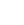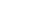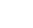CN/EN
CN/ENDecomposition of ammonia to produce hydrogen is the best choice for modern indus
Date：2014-06-25　From：Suzhou Captgas Gas Equipment Co., Ltd　Hits：407

Introduce the comparison of operating costs of three hydrogen production methods

1) The use of ammonia decomposition hydrogen production device and other hydrogen use methods

cost calculation:

①Operating cost of bottled hydrogen:

In general, the price of ordinary hydrogen in the market is 18 yuan/bottle, and the standard volume of a bottle of hydrogen is 6M3 under 15Mpa pressure. In fact, each bottle is only about 4.5M3. This calculation means that the price of ordinary hydrogen is about 4 yuan per cubic meter. .

The price of pure hydrogen on the market is 68 yuan per bottle, and if calculated per bottle, the price of pure hydrogen per cubic meter is about 15 yuan.

②The operating cost of water electrolysis hydrogen

In theory, producing 1 cubic meter of hydrogen by electrolysis requires 0.805KG of pure water and consumes 2.95KWH, but in reality, it requires 1kg of pure water and consumes 4.5-6KWH. This method consumes a lot of electricity and the purity of the hydrogen obtained is higher. Generally, the hydrogen content is 99.5-99.8%. For example, the operating cost of 1 kg of pure water is 1 yuan, and the price of 1KWH is 0.8 yuan. The calculated hydrogen price per cubic meter is between 4.5 and 6 yuan.

③Operation cost of ammonia decomposition of hydrogen:

Each kilogram of liquid ammonia can produce 2.78 standard cubic meters of mixed gas, the market price of liquid ammonia is 2,000 yuan per ton, and the liquid ammonia required for each cubic meter of ammonia decomposition gas is 0.72 yuan. The rated power of ammonia decomposition is generally 1KWH per cubic meter, but In fact, the electricity consumption per cubic meter of gas is about 40% of the rated power. For example, the price of 1KWH is 0.8 yuan, and the electricity consumption per cubic meter of ammonia decomposition gas is 0.8×40%=0.32. This calculates the operation of the mixed gas per cubic meter. The cost is 1.04 yuan.

for example:

According to your company's hydrogen consumption per hour is 25 M3/H, the 24-hour hydrogen consumption is 600 M3.

1. If you use ordinary bottle hydrogen, the price of ordinary bottle hydrogen is 4 yuan/M3 as shown above, and the total price of hydrogen per day is 600×4=2400 yuan.

2. If ammonia is used to decompose hydrogen, the price of hydrogen is 1.04 yuan, and the total price of hydrogen per day is 600×1.04=624 yuan.

In comparison, using ammonia to decompose hydrogen can save 2400-624=1776 yuan a day, and 1776×365 days=648,240 yuan a year. The price of 25 M3/H ammonia decomposition hydrogen production furnace with purification unit is 65,000 yuan. The investment in the liquid ammonia storage tank device is about 10,000 yuan, and the total investment is 75,000 yuan, so the investment can be recovered in one and a half months.

Note: The depreciation cost of the equipment is not considered in the ammonia decomposition hydrogen production.QQTelWechat